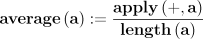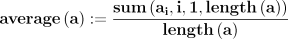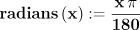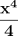Home | Mathematics | * Maxima | * Symbolic Mathematics Using Maxima 01. Acquiring, Installing and Testing 02. First Examples 03. Files and Functions 04. Creating Sets of Functions 05. Differential Equations 06. Fourier Analysis 07. A TeX Clipboard Daemon 08. Conclusion 09. TeX Clipboard Daemon Ruby ListingShare This Page
First Examples
P. Lutus Message Page

Preliminaries | More Substance | Moving On

(double-click any word to see its definition)

Preliminaries

Here are some useful hints for using wxMaxima and Maxima.

• To get help with a name used by Maxima, just type "? name":

? integrate
(wxMaxima launches a Maxima help dialog focused on the term "integrate")
? =
(sometimes the help finder doesn't match your entry exactly, but there will be a list of matches at the left to choose from)
• Maxima uses some nonintuitive syntax for assigning a value to a label. Here are the rules:

• Use ":" to assign a value to a variable: a : 3;, a : b; etc.
• Use "=" to compare values for equality: a = 3;,a = b; etc., also "<" (less than),">" (greater than) and "#" (unequal)
• Use ":=" to define a function: f(x) := x^2;
• Use "kill(name);" to erase a previous assignment of a variable or function: kill(a);, kill(f);
• Use "kill(all);" to erase all assignments.

Experiment with these assignment and comparison operators until you understand how they work:

a : 3; (spaces are optional, could be a:3;)
3
a
3
a + 3;
6
a * a;
9
f(x) := x^2;
f(x):=x2
f(x);
x2
f(9);
81
b : 4;
4
a - b;
-1
is(a = b); (think: "is a equal to b?")
false
is(a < b); (think: "is a less than b?")
true
is(a # b); (think: "is a not equal to b?")
true
kill(all); (this erases all our assignments)
done
is(a < b);
unknown

Hey! That was an intelligent answer! We erased all the assignments, so variables a and b are now undefined, so whether a < b (whether a is less than b) is ... well ... unknown. And the program knew it, and said so!

More Substance

Here is some simple arithmetic and algebra entered at the keyboard:

2^32
4294967296
111111111^2 (that's a row of nine 1s)
12345678987654321 (who would have guessed?)
sales_tax : 0.085 (that's 8.5%)
0.085
cost : 40 (the before-tax price of something)
40
cost * sales_tax (compute the tax on the cost)
3.4
cost * 1 + sales_tax (does this give the total amount?)
40.085 (apparently not)
cost * (1 + sales_tax) (does this give the total amount?)
43.4 (yes)

Notice about this example that the result depends on something called the "order of operations". In a mathematical expression without parentheses, there is an established order of operations:

1. Powers and roots.
2. Multiplication and division.

In that order.

To force a particular sequence of operations that differs from the natural order, use parentheses to group the desired operations together in your expressions. Operations inside parentheses are performed first, followed by operations outside parentheses. And there can be more than one layer of parentheses.

In this example:

((a+b)*(c+d))^n

First a is added to b, and c added to d. Then those two subgroups are multplied together. Then the result is raised to the power n. This example forces an opposite of the normal order of precedence on its terms. Remember when in doubt about how your expressions will be evaluated, group terms using parentheses to clarify your intentions.

Some more algebra examples:

%pi; (this is how we identify π to Maxima)
π (this will appear as a Greek letter only if a Greek font has been installed, see "Installation")
float(%pi); (meaning "give me the numerical value")
3.141592653589793
fpprec:1000; (increase the "floating point precision" or "fpprec")
1000
float(%pi); (again, "give me the numerical value")
3.141592653589793 (no change in the number of displayed places. Why?)
bfloat(%pi); ("bfloat" means "big float")
3.1415926535897932384626433832[943 digits]3001927876611195909216420196b0 (better, but not what I was hoping for)
set_display(ascii); (this is a special wxMaxima setting that forces all the digits to be printed out)
set_display(ascii);
bfloat(%pi); (try again)
(a list of 1000 digits of π)
set_display(xml);

The "fpprec" and "set_display(ascii)" settings only affect floating-point numbers, integers are always printed out fully:

50!;
30414093201713378043612608166064768844377641568960512000000000000
500!;
(a huge list of digits)

Here's an example that uses a Maxima function, plus one we define as well:

apply("+",[1,2,3,4,5]);
15

This gives me an idea.

average(a) := apply("+",a)/length(a);There is another way to write this function:

average(a) := sum(a[i],i,1,length(a))/length(a)average([1,2,3,4,5,6,7,8,9,10]);float(%);
5.5

Hold on. What does "%" mean? Well, it refers to the prior result. If you are creating intermediate results and want to refer to the immediate prior result, use "%". There are variations on this operator that can be used to get any arbitrary prior result — examine the help entry for "%".

Here's something we need to watch out for:

```set_display(ascii);
radians(x) := x * %pi / 180;
x %pi
180
```

Hold on again. Why did the display revert to a more primitive state (not drawing equations nicely or rendering Greek letters)? It's because we forgot to return to "set_display(xml)", so let's fix that:

set_display(xml);
set_display(xml);
radians(x) := x * %pi / 180;Okay, new rule: if you want to list all the digits of a floating-point value, use "set_display(ascii);". If you want wxMaxima to render equations and functions in a pretty way, use "set_display(xml);". It seems you can't have both at the same time.

? diff
(description of "diff" and related functions from the Maxima help file)
f(x) := x^3
f(x):=x3

According to my Calculus textbook, the first derivative of x3 should be 3 x2. Let's see.

diff(f(x),x)
3 x2
diff(f(x),x,2)
6 x
diff(f(x),x,3)
6
diff(f(x),x,4)
0

All correct. When using the "diff()" function, remember we can tell it which derivative to acquire by adding an optional third argument — 2 for second derivative, 3 for third derivative, etc.. Now let's try the function that does the opposite of derivation.

integrate(f(x),x);integrate(integrate(f(x),x),x);integrate(integrate(integrate(f(x),x),x),x);Okay, those are the right results, but it seems we can't simply specify which integral to obtain as we can with taking derivatives, instead we must nest our calls to "integrate". One could write a recursion function to do this to any arbitrary depth, but that's an advanced topic — later for that.

sum(n,n,1,9);
45
sum(n^2,n,1,9);
285

The function "sum()" adds together the results of an expression or function, according to the provided index values.

product(n,n,1,9);
362880
product(n^2,n,1,9);
131681894400

"product()" is somewhat like "sum()", but it multiplies the results together instead of adding them. Given how multiplication works, the above example might as well be:

product(n,n,2,9);
362880
product(n^2,n,2,9);
131681894400

Do you see why? It's because the original form multiplied the result by 1, a rather pointless operation.

Moving On

I ask that my readers feel free to explore what this program offers. Type in equations that you are curious about, create functions to encapsulate things you have discovered. Explore.

In the next section, I will show how to load and save your work, and how to put the solution to one problem in a wrapper that makes it available to solve others.

 Home | Mathematics | * Maxima | * Symbolic Mathematics Using Maxima 01. Acquiring, Installing and Testing 02. First Examples 03. Files and Functions 04. Creating Sets of Functions 05. Differential Equations 06. Fourier Analysis 07. A TeX Clipboard Daemon 08. Conclusion 09. TeX Clipboard Daemon Ruby ListingShare This Page